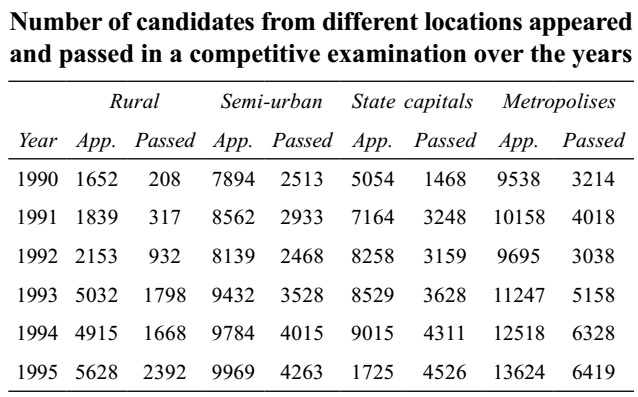## Introduction to Data Interpretation

#### Data Interpretation

Direction: Study the following table carefully and answer the question given below it.1. For the candidates from which of the following locations was there continuous increase both in appeared and passed?

1. As we can see in the graph that metropolises location has continuous increase both in appeared and passed.

##### Correct Option: D

As we can see in the graph that metropolises location has continuous increase both in appeared and passed.

1. In which of the following years was the percentage passed to appeared candidates from semi-urban area the least?

1. Our intelligent observation says that the required year cannot be 1993, 1994, 1995. Why? Because see the following conclusions:
% passed to appear = Passed / Appeared x 100
% of passed to appear is least when Passed / Appeared is the least or, Appeared / Passed is the most.

##### Correct Option: C

Our intelligent observation says that the required year cannot be 1993, 1994, 1995. Why? Because see the following conclusions:
% passed to appear = Passed / Appeared x 100
% of passed to appear is least when Passed / Appeared is the least or, Appeared / Passed is the most.
Now, we do the further calculations mentally. See the following conclusions:
For 1990: 7894 / 2513 ⇒ Quotient = 3 and Remainder ≈ 300
For 1991: 8562 / 2933 ⇒ Q = 3 and R ≈ 400
For 1992: 8139 / 2468 ⇒ Q = 3 and R ≈ 800
Similarly, for 1993; 1994, 1995, Q is 2.
So, 1992 gives the highest value.

1. What approximate value was the percentage drop in the number of semi-urban candidates appeared from 1991 to 1992?

1.  Percentage drop = Semi-urban candidates appeared in 1991 - Semi-urban candidates appeared in 1992 × 100 Number of semi-urban candidates appeared in 1991

##### Correct Option: A

 Percentage drop = Semi-urban candidates appeared in 1991 - Semi-urban candidates appeared in 1992 × 100 Number of semi-urban candidates appeared in 1991

 8562 - 8139 × 100 = 423 × 100 ≈ 42 × 10 = 5 % 8562 8562 84

1. In 1993 percentage of candidates passed to appeared was approximately 35 from which location?

1. We do not need to calculate the values for each year.
For Rural area : 35% of 5032 ≈ 35 × 50 = 1750 ≈ 1798
For Semi-urban area: 35% of 9500 ≈ 35 × 95 ≈ 3300

##### Correct Option: E

We do not need to calculate the values for each year.
For Rural area : 35% of 5032 ≈ 35 × 50 = 1750 ≈ 1798
For Semi-urban area : 35% of 9500 ≈ 35 × 95 ≈ 3300
Which cannot be approximated to 3500.
For State capitals : 35 × 85 = 3000
For Metropolises : 35 × 110 = 3850

1. The total number of candidates passed from Rural in 1993 and Semi-urban in 1990 was exactly equal to the total number of candidates passed from State capital in which of the following years?

1. The total number of candidates passed = Candidates passed from Rural in 1993 + Candidates passed from Semi-urban in 1990

##### Correct Option: C

The total number of candidates passed = Candidates passed from Rural in 1993 + Candidates passed from Semi-urban in 1990
The total number of candidates passed = 1798 + 2513 = 4311.
From the given table graph check the number of passed candidate from state capital is 4311 which is 1994.